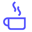a concise shot of dev knowledge
Become a Contributor
Concise shots of dev knowledge

RELATED TAGS

rsa
algorithm

# What is the RSA algorithm?Edpresso Team

The RSA algorithm is an asymmetric cryptography algorithm; this means that it uses a public key and a private key (i.e two different, mathematically linked keys). As their names suggest, a public key is shared publicly, while a private key is secret and must not be shared with anyone.

The RSA algorithm is named after those who invented it in 1978: Ron Rivest, Adi Shamir, and Leonard Adleman.

The following illustration highlights how asymmetric cryptography works:## How it works

The RSA algorithm ensures that the keys, in the above illustration, are as secure as possible. The following steps highlight how it works:

### 1. Generating the keys

1. Select two large prime numbers, $x$ and $y$. The prime numbers need to be large so that they will be difficult for someone to figure out.
2. Calculate $n = x$ x $y$.
3. Calculate the totient function; $\phi(n) = (x-1)(y-1)$.
4. Select an integer $e$, such that $e$ is co-prime to $\phi(n)$ and $1 < e < \phi(n)$. The pair of numbers $(n,e)$ makes up the public key.

Note: Two integers are co-prime if the only positive integer that divides them is 1.

1. Calculate $d$ such that $e.d = 1$ $mod$ $\phi(n)$.

$d$ can be found using the extended euclidean algorithm. The pair $(n,d)$ makes up the private key.

### 2. Encryption

Given a plaintext $P$, represented as a number, the ciphertext $C$ is calculated as:

$C = P^{e}$ $mod$ $n$.

### 3. Decryption

Using the private key $(n,d)$, the plaintext can be found using:

$P = C^{d}$ $mod$ $n$.

## Pseudocode

Consider an example of the RSA algorithm through the following pseudocode:

int x = 61, int y = 53;
int n = x * y;
// n = 3233.

// compute the totient, phi
int phi = (x-1)*(y-1);
// phi = 3120.

int e = findCoprime(phi);
// find an 'e' which is > 1 and is a co-prime of phi.
// e = 17 satisfies the current values.

// Using the extended euclidean algorithm, find 'd' which satisfies
// this equation:
d = (1 mod (phi))/e;
// d = 2753 for the example values.

public_key = (e=17, n=3233);
private_key = (d=2753, n=3233);

// Given the plaintext P=123, the ciphertext C is :
C = (123^17) % 3233 = 855;
// To decrypt the cypher text C:
P = (855^2753) % 3233 = 123;


RELATED TAGS

rsa
algorithm
RELATED COURSES

View all Courses

Keep Exploring

Learn in-demand tech skills in half the time